# Series- Test 2

1)

Find the missing figure in the series from the given answer figures.

[SSC CGL, 2004]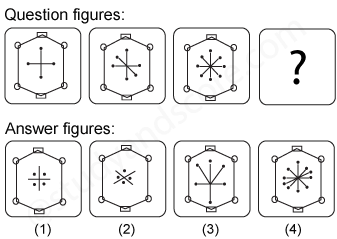2)

Find the missing figure in the series from the given answer figures.

[SSC CGL, 2004]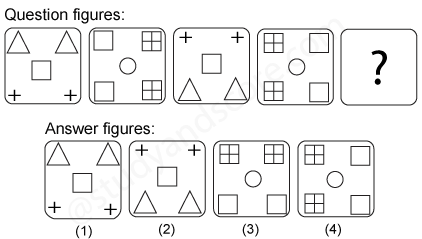3)

Find the missing figure in the series from the given answer figures.

[SSC CGL, 2004]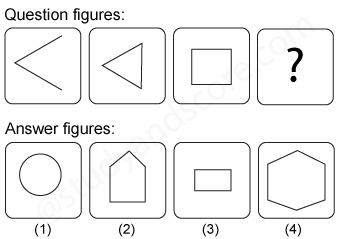4)

Find the missing figure in the series from the given answer figures.

[SSC CGL, 2004]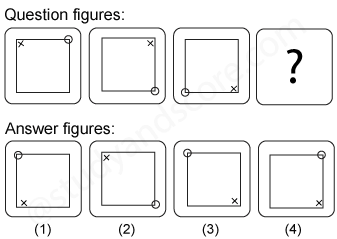5)

Find the missing figure in the series from the given answer figures.

[SSC CGL, 2004]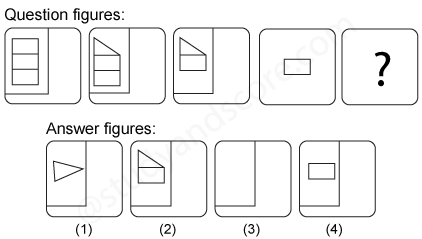--Share with your friends --